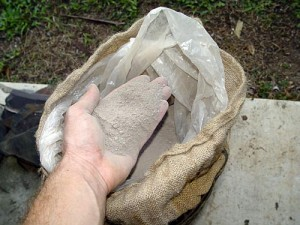﻿ Fire Clay 1 cubic centimeter volume to grams converter

# fire clay conversion

## Amount: 1 cubic centimeter (cc - cm3) of volume Equals: 1.30 grams (g) in mass

Converting cubic centimeter to grams value in the fire clay units scale.

TOGGLE :   from grams into cubic centimeters in the other way around.

## fire clay from cubic centimeter to gram Conversion Results:

### Enter a New cubic centimeter Amount of fire clay to Convert From

* Whole numbers, decimals or fractions (ie: 6, 5.33, 17 3/8)
* Precision is how many numbers after decimal point (1 - 9)

Enter Amount :
Decimal Precision :

CONVERT :   between other fire clay measuring units - complete list.

Conversion calculator for webmasters.

## Fire clay mass vs. volume

Powdered in dry-loose form (not packed) fireclay, or fire clay, as it comes out from a purchased bag, has quite a high mass of 1303 grams per 1000 cc - cm3 - 1 liter - 61.024 cubic inches, 61 cu in rounded. This makes it 1.303g/cm3 or 0.753oz/cu-in density.That is for how heavy a raw fire clay is. No wonder the bags feel so excruciatingly heavy.

For more comprehensive information on fire clay, and its uses in heat resistant applications, see the what is fire clay. The page also talks about how and where to obtain the right fire clay from the nature (if needed to sell it or how to go about making inexpensive raw clay adobe tiles and mud ovens out if it.)

Convert fire clay measuring units between cubic centimeter (cc - cm3) and grams (g) but in the other reverse direction from grams into cubic centimeters.

 conversion result for fire clay: From Symbol Equals Result To Symbol 1 cubic centimeter cc - cm3 = 1.30 grams g

# Converter type: fire clay measurements

This online fire clay from cc - cm3 into g converter is a handy tool not just for certified or experienced professionals.

First unit: cubic centimeter (cc - cm3) is used for measuring volume.
Second: gram (g) is unit of mass.

## fire clay per 1.30 g is equivalent to 1 what?

The grams amount 1.30 g converts into 1 cc - cm3, one cubic centimeter. It is the EQUAL fire clay volume value of 1 cubic centimeter but in the grams mass unit alternative.

How to convert 2 cubic centimeters (cc - cm3) of fire clay into grams (g)? Is there a calculation formula?

First divide the two units variables. Then multiply the result by 2 - for example:
1.303 * 2 (or divide it by / 0.5)

QUESTION:
1 cc - cm3 of fire clay = ? g

1 cc - cm3 = 1.30 g of fire clay

## Other applications for fire clay units calculator ...

With the above mentioned two-units calculating service it provides, this fire clay converter proved to be useful also as an online tool for:
1. practicing cubic centimeters and grams of fire clay ( cc - cm3 vs. g ) measuring values exchange.
2. fire clay amounts conversion factors - between numerous unit pairs.
3. working with - how heavy is fire clay - values and properties.

International unit symbols for these two fire clay measurements are:

Abbreviation or prefix ( abbr. short brevis ), unit symbol, for cubic centimeter is:
cc - cm3
Abbreviation or prefix ( abbr. ) brevis - short unit symbol for gram is:
g

### One cubic centimeter of fire clay converted to gram equals to 1.30 g

How many grams of fire clay are in 1 cubic centimeter? The answer is: The change of 1 cc - cm3 ( cubic centimeter ) unit of fire clay measure equals = to 1.30 g ( gram ) as the equivalent measure for the same fire clay type.

In principle with any measuring task, switched on professional people always ensure, and their success depends on, they get the most precise conversion results everywhere and every-time. Not only whenever possible, it's always so. Often having only a good idea ( or more ideas ) might not be perfect nor good enough solution. If there is an exact known measure in cc - cm3 - cubic centimeters for fire clay amount, the rule is that the cubic centimeter number gets converted into g - grams or any other fire clay unit absolutely exactly.

Conversion for how many grams ( g ) of fire clay are contained in a cubic centimeter ( 1 cc - cm3 ). Or, how much in grams of fire clay is in 1 cubic centimeter? To link to this fire clay cubic centimeter to grams online converter simply cut and paste the following.
The link to this tool will appear as: fire clay from cubic centimeter (cc - cm3) to grams (g) conversion.

I've done my best to build this site for you- Please send feedback to let me know how you enjoyed visiting.